##### Actions

The branch of number theory dealing with problems involving the partitioning (or decomposition) of integers into summands of a given type, and also with the algebraic and geometric analogues of such problems concerning algebraic number fields and sets of lattice points. These problems are said to be additive, and the ones usually considered are partition problems of large integers.

Classical problems in additive number theory include: the representation of a number as a sum of four squares, nine cubes, etc. (cf. Waring problem); the representation of a given number as a sum of not more than three prime numbers (cf. Goldbach problem); and the representation of a given number as a sum of one prime number and two squares (cf. Hardy–Littlewood problem). Problems in additive number theory are solved by analytic, algebraic, elementary and mixed methods, and also by methods based on probabilistic concepts. Depending on the method selected, additive problems form a part of various branches of number theory — analytic, algebraic and probabilistic number theory.

The first systematic results in this field were obtained in 1748 by L. Euler, who employed power series to study the partition of integers into positive summands; in particular, he investigated the problem of partitioning a given number into a given number of summands.

Many classical problems in additive number theory are solved by means of generating functions. This method was introduced by Euler and forms the basis of the analytic methods developed by G.H. Hardy, J.E. Littlewood and I.M. Vinogradov. As a first step, assign to given sequences(where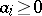is an integer, and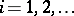) the power series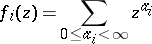and consider the corresponding generating function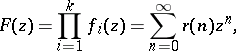where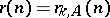is the number of representations of the numberin the formHere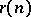can be calculated using the Cauchy integral. In Vinogradov's method trigonometric sums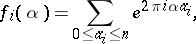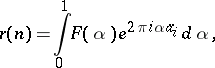are used instead of power series. A main part of, located in intervals distributed over neighbourhoods of rational points, is extracted. Instead of the analytic properties ofwhich, in several problems of additive number theory, necessitate the use of hypotheses related to the Riemann hypothesis (cf. Riemann hypotheses), a crucial role in the calculation ofis played by purely arithmetic estimates of trigonometric sums by Vinogradov's method, and by the law of the distribution of prime numbers in arithmetic progressions, obtained by the analytic methods of the theory of Dirichlet-functions. It was found that, depending on, either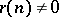for all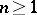orfor sufficiently large(), or the relationis valid for almost all values of, i.e.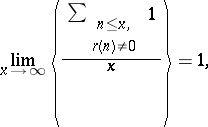or, finally,can be expressed by an asymptotic formula. The smallest numbersatisfying one of the properties above is denoted by, or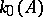, respectively. If, where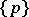is the sequence of prime numbers, and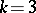, then one obtains Vinogradov's theorem: Every sufficiently large odd integer can be represented as a sum of three prime numbers; if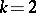, one obtains Chudakov's theorem: Almost all even integers can be represented as a sum of two prime numbers.

Certain problems in additive number theory are solved by studying the structure of the sets obtained by addition of sequences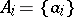given only in terms of their densities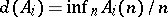, where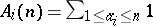. If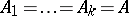, then the positivity of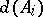implies that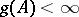. The application of this result to problems in additive number theory in which sequences of density zero are added, is realized by constructing a new sequence, with a positive density, from the given sequence. This is done, mainly, by sieve methods (cf. Sieve method), by which it can be proved thatis positive. It was shown by L.G. Shnirel'man in this way that positive integers can be represented as a sum of a bounded number of prime summands, while Yu.V. Linnik found an elementary solution of Waring's problem.

The elementary sieve methods of V. Brun (cf. Brun sieve) and of A. Selberg (cf. Selberg sieve), if applied to a number of problems in additive number theory, yield results which are as yet unobtainable by analytic tools. However, the most advanced solutions of certain problems in additive number theory are obtained by combining analytic and elementary methods. In sieve methods, the principle of sieving the prime numbers out of the sequence of positive integers (cf. Eratosthenes, sieve of) is extended to other sequences as well. Thus, a simultaneous, suitably accurate, sieving of the prime numbers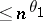and, respectively (whereand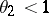are suitably chosen positive constants) out of the sequences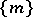and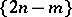yields a solution of the so-called Goldbach–Euler quasi-problem on the representation of an even integer as a sum of two numbers one of which has at most, while the other has at most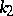prime factors.

Linnik in 1959 successfully solved the Hardy–Littlewood problem by the dispersion method developed by him. He showed  that any sufficiently large integer can be represented as the sum of a prime number and two squares of integers. The dispersion method yielded solutions of several so-called binary problems, viz. how to find the number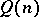of solutions of the equation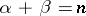, whereandrun through given sequences which are sufficiently well distributed in arithmetic progressions. Linnik's method involves the use of elementary concepts of probability theory, which were also employed by P.L. Chebyshev in his proof of the law of large numbers. It consists of reducing the given binary equation to a large number of auxiliary equations for which the expected number of solutions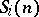and the true numbers of solutions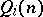of the equations are connected. If it is shown by a computation of the dispersion thatdoes not differ much from"in the mean" , then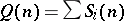(within an acceptable error). The dispersion method was also employed in the study of the general Hardy–Littlewood equation.

The range of application of the dispersion method intersects with the range of application of the method of the large sieve, developed in 1941 by Linnik. The method makes it possible to sieve out sequences with the aid of prime numbers, with an increasing number of discarded residues. Actually, the method of the large sieve is a consequence of the laws of distribution of weakly-dependent random variables.

Additive number theory includes problems whose systematic study belongs to other branches of number theory: the problem of representing integers by quadratic or higher-degree expressions; and the study of Diophantine equations, which may be treated in the framework of general additive number theory.

In modern number theory various branches of additive number theory are now being intensively developed. There is a trend to transfer the problems and methods of additive number theory to arbitrary algebraic number fields.